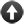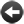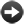§22.5. Map, filter and reduce

When a mass of computations has to be done, the traditional approach is to work through them in a "repeat" loop. One modern alternative, growing in popularity, is to form a list of inputs; then apply the same computation to each input in turn to form a list of results (this is called "mapping"); throw out any bad or unwanted results ("filtering"); and then combine the surviving results into a single composite answer ("reducing", though some programming languages call this "folding" or "accumulation"; it's a much-reinvented idea).

Inform provides all three of these fundamental list-processing operations. There is no special term for a "map", because Inform treats it as another case of "applied to".

(phrase value -> value) applied to (list of values) ... value

This phrase takes the list, applies the phrase to each entry in the list, and forms a new list of the result. Example:

To decide what number is double (N - a number) (this is doubling):
decide on N plus N.

Then "doubling applied to 2" produces 4, by the simpler definition of "applied to", but also:

doubling applied to {2, 3, 4}

produces the list {4, 6, 8}.

More divertingly, suppose we define:

To decide what text is the longhand form of (N - a number)
(this is spelling out):
decide on "[N in words]".

To decide what text is the consonant form of (T - text)
(this is txtng):
replace the regular expression "<aeiou>" in T with "";
decide on T.

Then we can write a chain of three maps in succession:

txtng applied to spelling out applied to doubling applied to {3, 8, 4, 19, 7}

to produce the value {"sx", "sxtn", "ght", "thrty-ght", "frtn"}.

Next, filtering. Here we make use of descriptions, in order to say what values will be allowed through the filter. So:

filter to (description of values) of (list of values) ... value

This phrase produces a new list which is a thinner version of the one given, so that it contains only those values which match the description given. Example:

filter to even numbers of {3, 8, 4, 19, 7}

produces {8, 4}, with the values 3, 19, and 7 failing to make it through. A sufficiently fine filter may well thin out a list to a single entry, or even no entries at all, but the result is always a list.

To get the full effect of filtering, we probably need to define an adjective or two. For example:

Definition: a text (called T) is lengthy if the number of characters in it is greater than 6.

We can then write, for example:

let L be the filter to lengthy texts of spelling out applied to {15, 2, 20, 29, -4};
showme L;

which produces the list {"fifteen", "twenty-nine", "minus four"}.

Lastly, reduction. In order to combine a whole list of values, we need a phrase to combine any two. Here are some samples:

To decide what number is the larger of (N - number) and (M - number)
(this is maximization):
if N > M, decide on N;
decide on M.

To decide what text is the concatenation of (X - text) and (Y - text)
(this is concatenation):
decide on "[X][Y]".

And here are some sample reductions:

let X be the maximization reduction of {3, 8, 4, 19, 7};
let Y be the concatenation reduction of txtng applied to spelling out
applied to doubling applied to {3, 8, 4, 19, 7};

sets X to 19, the highest of the values, and Y to the text "sxsxtnghtthrty-ghtfrtn". In each case a list has been reduced to a single value which somehow combines the contents.

(phrase (value, value) -> value) reduction of (list of values) ... value

This phrase works through the list and accumulates the values in it, using the phrase supplied. Example: if we have

To decide what number is the sum of (N - number) and (M - number)
(this is summing):
decide on N + M.

then the summing reduction of {3, 8, 4, 19, 7} is the number 41, obtained by

(((3 + 8) + 4) + 19) + 7

so that the summing phrase has been used four times.

Is map/filter/reduce always a good idea? Devotees point out that almost any computation can be thought of in this way, and in systems where the work has to be distributed around multiple processors it can be a very powerful tool. (There are programming languages without loops where it's essentially the only tool.) At its best, it reads very elegantly: one assembles all of the tools needed - definitions of doubling, lengthy, spelling out, concatenation and so on - and then each actual task is expressed in a single line at the end.

On the other hand, there are also times when this is a needlessly complicated disguise for what could more easily be done with a "repeat" loop, and also more efficiently since assembling and dismantling lists in memory does take some overhead time. So these list operations are not a panacea, but it's good to have them available.Start of Chapter 22: Advanced PhrasesBack to §22.4. Default values for phrase kindsOnward to §22.6. Generic phrases